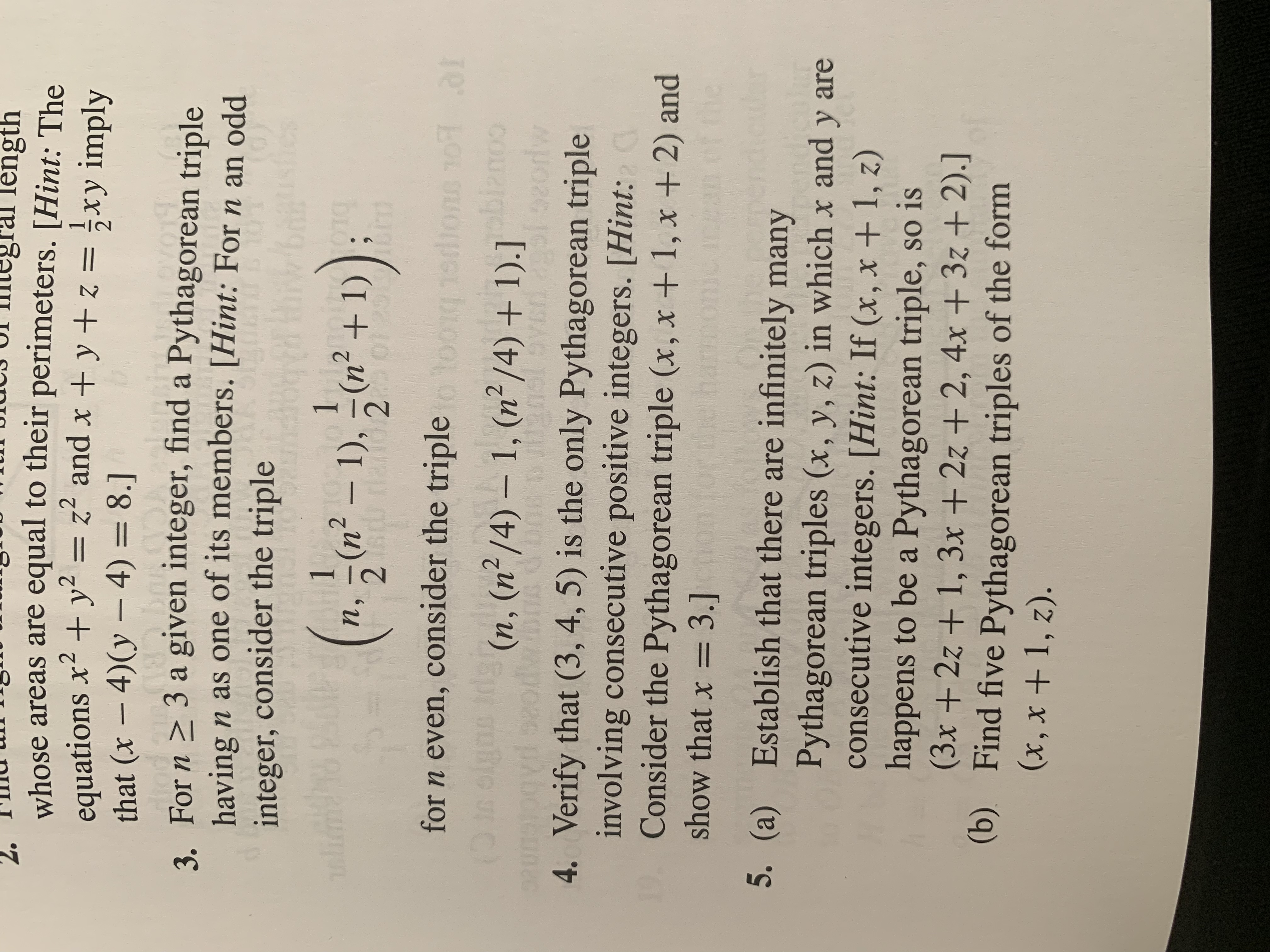# length whose areas are equal to their perimeters. [Hint: The equations x2y that (x 4)(y-4) 8.] 3. For n 3 a given integer, find a Pythagorean triple having n as one of its members. [Hint: For n an odd integer, consider the triple = z and x + y +z xy imply 1 1 (n21) 2 (n 1) п, 2 0129 16E for n even, consider the triple toom Jons ol al 10R (D OLB S (n, (n2/4) - 1, (n2/4) + 1).] do8 Dilenál ozs pb 03 oorlw 4. Verify that (3, 4, 5) is the only Pythagorean triple involving consecutive positive integers. [Hint: Consider the Pythagorean triple (x, x + 1, x +2) and show that x = 3.] mo 5. (a) Establish that there are infinitely many Pythagorean triples (x, y, z) in which x and y are consecutive integers. [Hint: If (x, x + 1, z) happens to be a Pythagorean triple, so is (3x +2z + 1, 3x + 2z + 2, 4x + 3z + 2).] (b) Find five Pythagorean triples of the form (x, x1, z).

Questionhelp_outlineImage Transcriptioncloselength whose areas are equal to their perimeters. [Hint: The equations x2y that (x 4)(y-4) 8.] 3. For n 3 a given integer, find a Pythagorean triple having n as one of its members. [Hint: For n an odd integer, consider the triple = z and x + y +z xy imply 1 1 (n21) 2 (n 1) п, 2 0129 16E for n even, consider the triple toom Jons ol al 10R (D OLB S (n, (n2/4) - 1, (n2/4) + 1).] do8 Dilenál ozs pb 03 oorlw 4. Verify that (3, 4, 5) is the only Pythagorean triple involving consecutive positive integers. [Hint: Consider the Pythagorean triple (x, x + 1, x +2) and show that x = 3.] mo 5. (a) Establish that there are infinitely many Pythagorean triples (x, y, z) in which x and y are consecutive integers. [Hint: If (x, x + 1, z) happens to be a Pythagorean triple, so is (3x +2z + 1, 3x + 2z + 2, 4x + 3z + 2).] (b) Find five Pythagorean triples of the form (x, x1, z). fullscreen